# idopNetwork_vignette

#### 11/21/2022

library(idopNetwork)
backup_options <- options()
test_result = idopNetwork:::test_result

idopNetwork is packed as a cartographic tool that performs power curve fitting, classification, variable selection, microbial abundance decomposition, and network visualization based on microbial 16S rRNA gene sequencing metadata.

For complete details on the use and execution of this protocol, please refer to Chen et al and Cao et al.

# 1. Input data

Before running idopNetwork, user need to provide datasets, and they should be cleaned and merged to exactly the same format of the example data.

## 1.1 gut microbe OTUs data

Microbe Operational taxonomic unit dataset must have first column as IDs for microbes.

data("gut_microbe")
View(gut_microbe)
name GQ_UC1 GQ_UC3 GQ_UC4 GQ_UC5
Escherichia_coli 176 1701 28506 177
Arthrobacter_oxydans 3 215 1578 1
Ruminococcus_gnavus 744 0 4 733
Bacteroides_plebeius 271 4401 7 8394
Bacteroides_pyogenes 0 15 0 0
Clostridium_tertium 305 1 1 270
Bacteroides_stercoris 24 0 0 11
Flavisolibacter_ginsengisoli 0 123 831 0
Bacteroides_massiliensis 0 6098 6 0
Prevotella_heparinolytica 0 6 0 0

## 1.2 mustard microbe OTUs data

data("mustard_microbe")
View(mustard_microbe)
ID 8_0149.leaf 8_0157.leaf 8_0158.leaf 8_0160.leaf
2 OTU_1 3 2 59 268
3 OTU_2 8 13 1890 44
4 OTU_3 5485 3302 4522 6581
5 OTU_4 42 351 7 4
6 OTU_5 16 2002 24 43
7 OTU_6 1645 1170 10636 31686
8 OTU_7 4050 4094 13 66
9 OTU_8 6 282 124 27
10 OTU_9 102 5 10 18
11 OTU_10 25 1393 35 19
JAM_E4.leaf 8_0149.root 8_0157.root 8_0158.root 8_0160.root
2 7 28861 122 1 9
3 4 11618 19 14 1894
4 4981 481 755 17914 15998
5 622 862 1072 11 103
6 10014 447 758 19 289
7 577 1010 490 25030 29443
8 2233 13 5575 26 64
9 19 3348 36 33 46
10 362 6269 228 2 459
11 3071 4 6454 6 234

# 2. power curve fitting

The first major step in our idopNetwork reconstruction is to fit allometric growth curves to the data using the power function. This is easily done by using the related function power_fit. This function needs cleaned dataset as input and will return fitted OTUs for given dataset. Then the fitted output with original dataset can be transfer into function power_equation_plot for quick visualization

df = data_cleaning(gut_microbe)
result1 = power_equation_fit(df)
power_equation_plot(result1)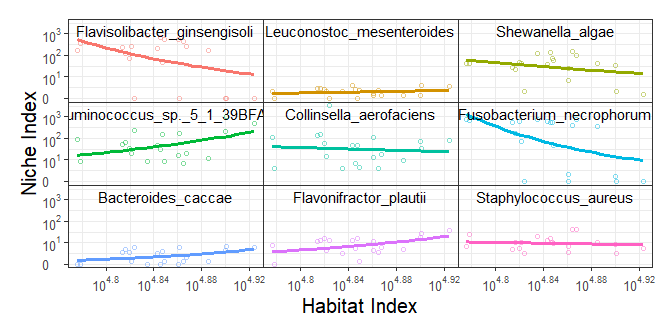# 3. Functional clustering

In this step we implement the power equation into functional clustering to detect different microbial modules. If after clustering there are still too many microbes within a certain module for network reconstruction, we can rerun functional clustering to further classify this module into distinct submodules.

## 3.1 mean curve

we fit mean vector of each cluster center by power equation(assume k=3)

matplot(t(power_equation(x = 1:30, matrix(c(2,1,3,0.2,0.5,-0.5),nrow = 3, ncol = 2))),
type = "l",
xlab = "time",
ylab = "population")
legend("topright",
c("cluster 1", "cluster 2", "cluster 3"),
lty = c(1,2,3),
col = c(1,2,3),
box.lwd = 0)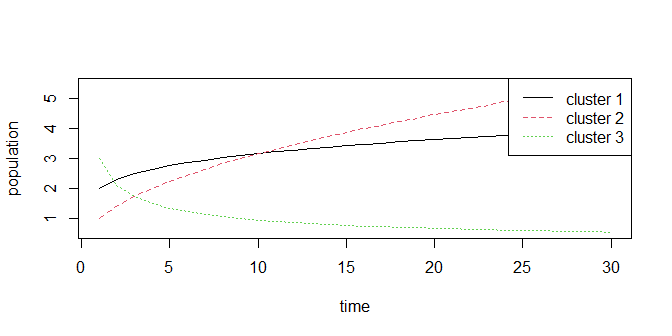## 3.2 covariance matrix

we fit covariance matrix of multivariate normal distribution with SAD1, it can be showed as

get_SAD1_covmatrix(c(2,0.5), n = 5)
#>      [,1]  [,2]  [,3]  [,4]  [,5]
#> [1,] 0.25  0.50  1.00  2.00  4.00
#> [2,] 0.50  1.25  2.50  5.00 10.00
#> [3,] 1.00  2.50  5.25 10.50 21.00
#> [4,] 2.00  5.00 10.50 21.25 42.50
#> [5,] 4.00 10.00 21.00 42.50 85.25

## 3.3 initial parameters

we can check initial parameters (k=4)

get_par_int(X = log10(df+1), k = 4, times = as.numeric(log10(colSums(df)+1)))
#> Error in nls(y ~ a * x^b, start = list(a = a, b = b), control = nls.control(maxiter = 1000,  :
#>   number of iterations exceeded maximum of 1000
#> $initial_cov_params #>  0.50000 1.09618 #> #>$initial_mu_params
#>                 a           b
#> [1,] 1.222824e+15 -21.7298738
#> [2,] 1.275764e+01  -0.8462021
#> [3,] 2.241051e-35  50.4618421
#> [4,] 1.931704e+02  -3.6050446
#>
#> $initial_probibality #> #> 1 2 3 4 #> 0.24590164 0.06557377 0.27868852 0.40983607 #use kmeans to get initial centers tmp = kmeans(log10(df+1),4)$centers
tmp2 = power_equation_fit(tmp, trans = NULL)
power_equation_plot(tmp2, label = NULL,n = 4)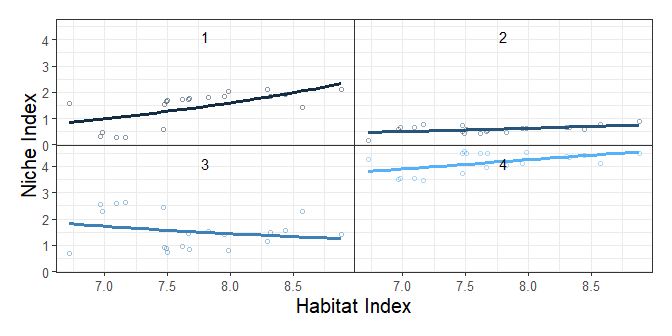idopNetowrk already wrapped the mean curve modelling, covariance matrix modelling and likelihood ratio calculation into a function fun_clu().

options(max.print = 10)
fun_clu(result1$original_data, k = 3, iter.max = 5) #> initial value 1222.767913 #> final value 1117.320216 #> converged #> #> iter = 1 #> Log-Likelihood = 1117.254 #> initial value 1112.059734 #> iter 10 value 1109.958326 #> iter 20 value 1100.656602 #> iter 30 value 1100.608350 #> iter 40 value 1100.531652 #> final value 1100.524918 #> converged #> #> iter = 2 #> Log-Likelihood = 1100.522 #> initial value 1097.775112 #> final value 1097.703356 #> converged #> #> iter = 3 #> Log-Likelihood = 1097.696 #> initial value 1097.692569 #> final value 1097.268152 #> converged #> #> iter = 4 #> Log-Likelihood = 1097.265 #> initial value 1097.205439 #> final value 1097.205439 #> converged #> #> iter = 5 #> Log-Likelihood = 1097.207 #> initial value 1097.205306 #> final value 1097.205306 #> converged #> #> iter = 6 #> Log-Likelihood = 1097.204 #>$cluster_number
#>  3
#>
#> $Log_likelihodd #>  1097.204 #> #>$AIC
#>  2214.409
#>
#> $BIC #>  2233.121 #> #>$cov_par
#>  0.3226165 0.7364701
#>
#> $mu_par #> [,1] [,2] #> [1,] 1.329923e+14 -20.272606 #> [2,] 2.566307e-13 18.282426 #> [3,] 1.065010e+03 -3.519009 #> #>$probibality
#>  0.35045898 0.60787434 0.04166668
#>
#> $omega #> [,1] [,2] [,3] #> [1,] 9.996019e-01 3.981430e-04 3.220609e-23 #> [2,] 9.900970e-01 9.902998e-03 5.222845e-24 #> [3,] 2.325020e-01 7.674980e-01 3.060533e-34 #> [ reached getOption("max.print") -- omitted 45 rows ] #> #>$cluster
#>      MC_UC3 MC_UC4 YZJC_UC3 GQ_UC3 ZC_UC3 MC_UC2 GQ_UC1 GQ_UC4 HJC_UC3 ZC_UC4
#>      HJC_UC1 HJC_UC4 HC_UC2 ZC_UC1 HC_UC4 JJC_UC2 HJC_UC2 MC_UC1 GQ_UC5 ZC_UC5
#>      apply(omega, 1, which.max)
#>  [ reached 'max' / getOption("max.print") -- omitted 48 rows ]
#>
#> $cluster2 #> MC_UC3 MC_UC4 YZJC_UC3 GQ_UC3 ZC_UC3 MC_UC2 GQ_UC1 GQ_UC4 HJC_UC3 ZC_UC4 #> HJC_UC1 HJC_UC4 HC_UC2 ZC_UC1 HC_UC4 JJC_UC2 HJC_UC2 MC_UC1 GQ_UC5 ZC_UC5 #> apply(omega, 1, which.max) #> [ reached 'max' / getOption("max.print") -- omitted 48 rows ] #> #> [ reached getOption("max.print") -- omitted 2 entries ] Usually we use multithread to calcuation k = 2-n and then to decide best k , fun_clu_BIC uses BIC to select best cluster number by default. result2 = fun_clu_parallel(result1$original_data, start = 2, end = 5)
best.k = which.min(sapply( result2 , "[[" , 'BIC' )) + 1 #skipped k = 1
best.k
#>  7

fun_clu_BIC(result = result2)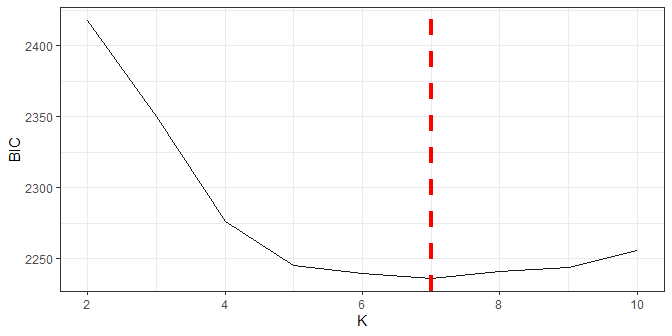#we can direct give other k value
fun_clu_plot(result = result2, best.k = best.k)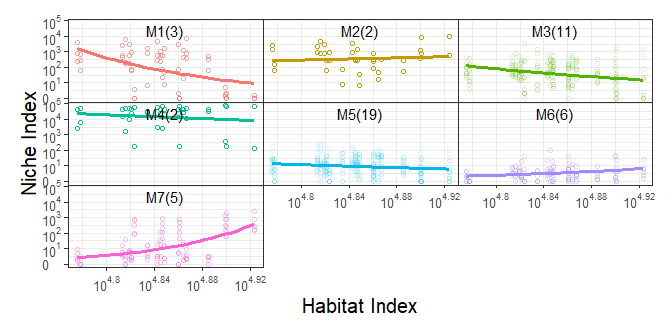In this part we select part of mustard data for demonstration purpose.

data("mustard_microbe")
df2 = data_cleaning(mustard_microbe, x = 160)
res_l = power_equation_fit(df2[,1:5]
res_r = power_equation_fit(df2[,89:95])
res1 = data_match(result1 = res_l, result2 = res_r)
res2 = bifun_clu_parallel(data1 = res1$dataset1$original_data,
data2 = res1$dataset2$original_data,
Time1 = res1$dataset1$Time,
Time2 = res1$dataset2$Time,
start = 2,
end = 10,
iter.max = 10)
res2 = bifun_clu_parallel(data1 = res1$dataset1$original_data,
data2 = res1$dataset2$original_data,
Time1 = res1$dataset1$Time,
Time2 = res1$dataset2$Time,
start = 2,
end = 10,
iter.max = 10)
fun_clu_BIC(result = res2)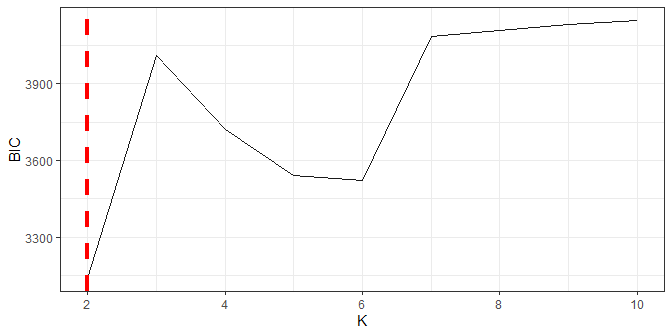#we can set best.k directly
bifun_clu_plot(result = res2, best.k = 3, color1 = "#C060A1", color2 = "#59C1BD")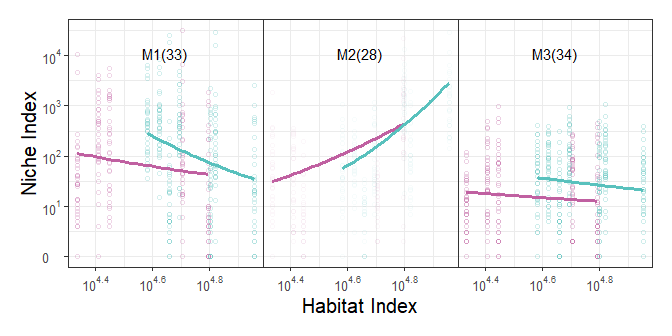## 3.6 sub-clustering

Sometimes a module is still too large for network reconstruction, which is determined by Dunbar’s number, we can further cluster it into sub-modules.

res3 = bifun_clu_convert(res2, best.k = 3)
large.module = order(sapply(res3$a$Module.all,nrow))

res_suba = fun_clu_select(result_fit = res1$dataset1, result_funclu = res3$a, i = large.module)
res_subb = fun_clu_select(result_fit = res1$dataset2, result_funclu = res3$b, i = large.module)
dfsuba_l = power_equation_fit(res_suba$original_data) dfsubb_r = power_equation_fit(res_subb$original_data)
ressub1 = data_match(result1 = dfsuba_l, result2 = dfsubb_r)
ressub2 = bifun_clu_parallel(data1 = ressub1$dataset1$original_data,
data2 = ressub1$dataset2$original_data,
Time1 = ressub1$dataset1$Time,
Time2 = ressub1$dataset2$Time,
start = 2,
end = 5,
iter.max = 3)
ressub2 = test_result$d2_subcluster fun_clu_BIC(result = ressub2)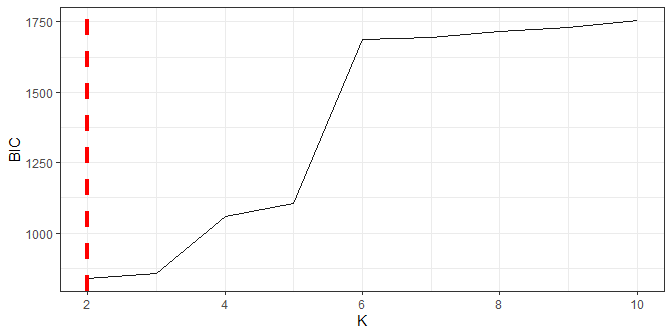bifun_clu_plot(result = ressub2, best.k = 2, color1 = "#C060A1", color2 = "#59C1BD", degree = 1)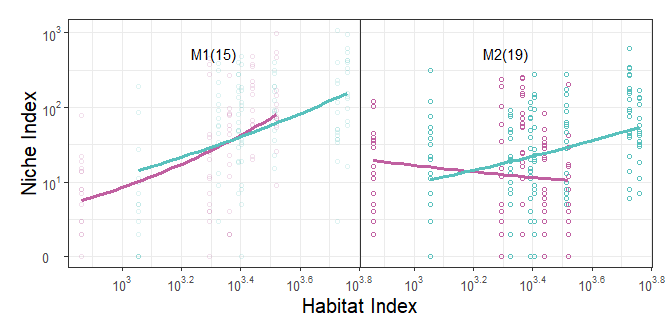# 4. LASSO-based variable selection idopNetwork implements a LASSO-based procedure to choose a small set of the most significant microbes/module that links with a given microbes/modules. get_interaction()return a compound list contain the target microbe/module name, the most relevant Modules/microbes names and the coefficients. ## 4.1 For Modules result3 = fun_clu_convert(result2,best.k = best.k) df.module = result3$original_data
get_interaction(df.module,1)
#> $ind.name #>  "M1" #> #>$dep.name
#>  "M5"
#>
#> $coefficient #>  5.982483 ## 4.2 For Microbes #we can the microbial relationship in Module1 df.M1 = result3$Module.all$1 get_interaction(df.M1,1) #> Warning: Option grouped=FALSE enforced in cv.glmnet, since < 3 observations per #> fold #> Warning: Option grouped=FALSE enforced in cv.glmnet, since < 3 observations per #> fold #> Warning: Option grouped=FALSE enforced in cv.glmnet, since < 3 observations per #> fold #>$ind.name
#>  "Arthrobacter_oxydans"
#>
#> $dep.name #>  "Fusobacterium_necrophorum" #> #>$coefficient
#>  0.08263525

# 5 qdODE solving

qdODE system is build based on variable selection results, it has unique ability to decompose the observed module/microbe abundance level into its independent component and dependent component, which can be used for inferring idopNetwork.

## 5.1 solving qdODE between modules

options(max.print = 10)
# first we test solving a qdODE
module.relationship = lapply(1:best.k, function(c)get_interaction(df.module,c))
ode.test = qdODE_all(result = result3, relationship = module.relationship, 1, maxit = 100)
#> iter   10 value 0.004432
#> final  value 0.000002
#> converged
# we can view the result
qdODE_plot_base(ode.test)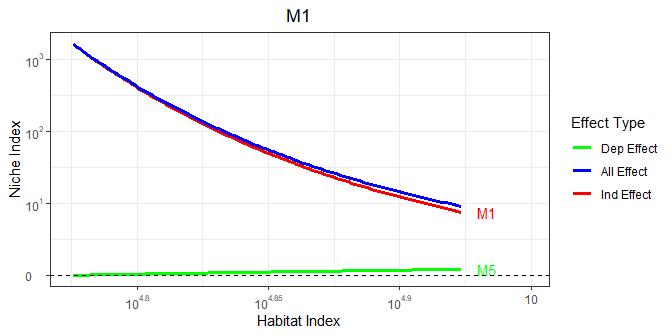# then we solve all qdODEs
ode.module = qdODE_parallel(result3) 
qdODE_plot_all(ode.module)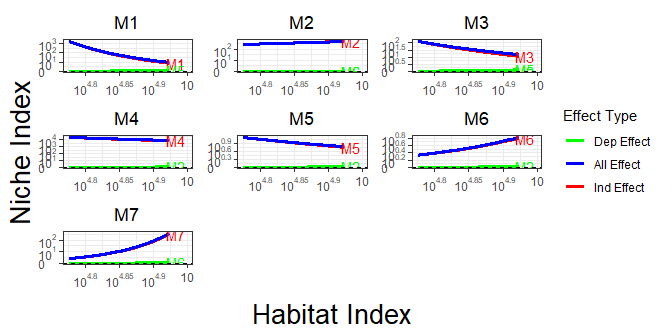## 5.2 solving qdODE within a module

result_m1 = fun_clu_select(result_fit = result1, result_funclu = result3, i = 1)
ode.M1 = qdODE_parallel(result_m1)
qdODE_plot_all(ode.M1)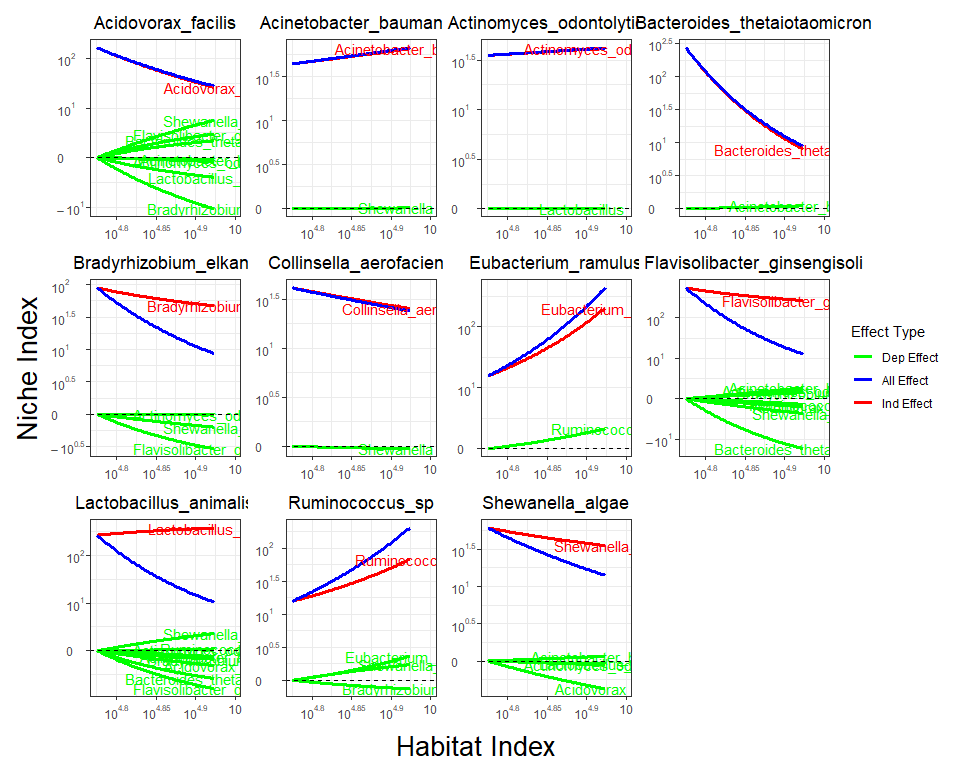# 6 idopNetwork reconstruction

The final step of this guide is to visualization the multilayer network, and our package provide network_plot function to easily draw our idopNetwork. We can simply plug previous qdODE results into network_conversion function, and it will convert qdODE result for network visualization

net_module = lapply(ode.module$ode_result, network_conversion) network_plot(net_module, title = "Module Network")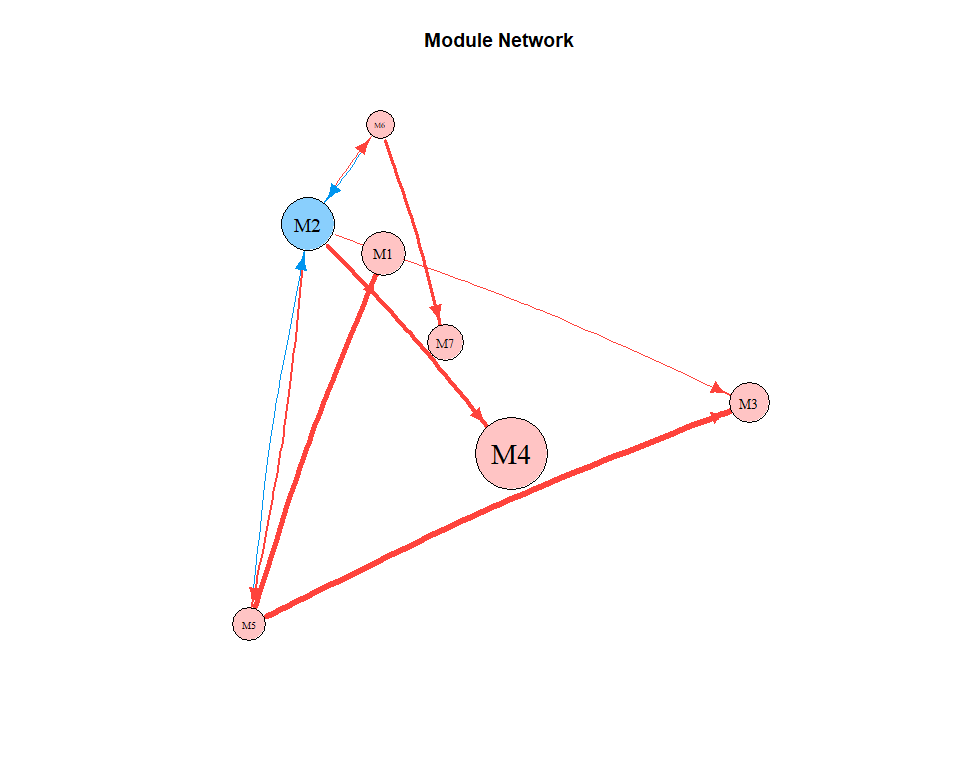## 6.2 network within a module net_m1 = lapply(ode.M1$ode_result, network_conversion)
network_plot(net_m1, title = "M1 Network")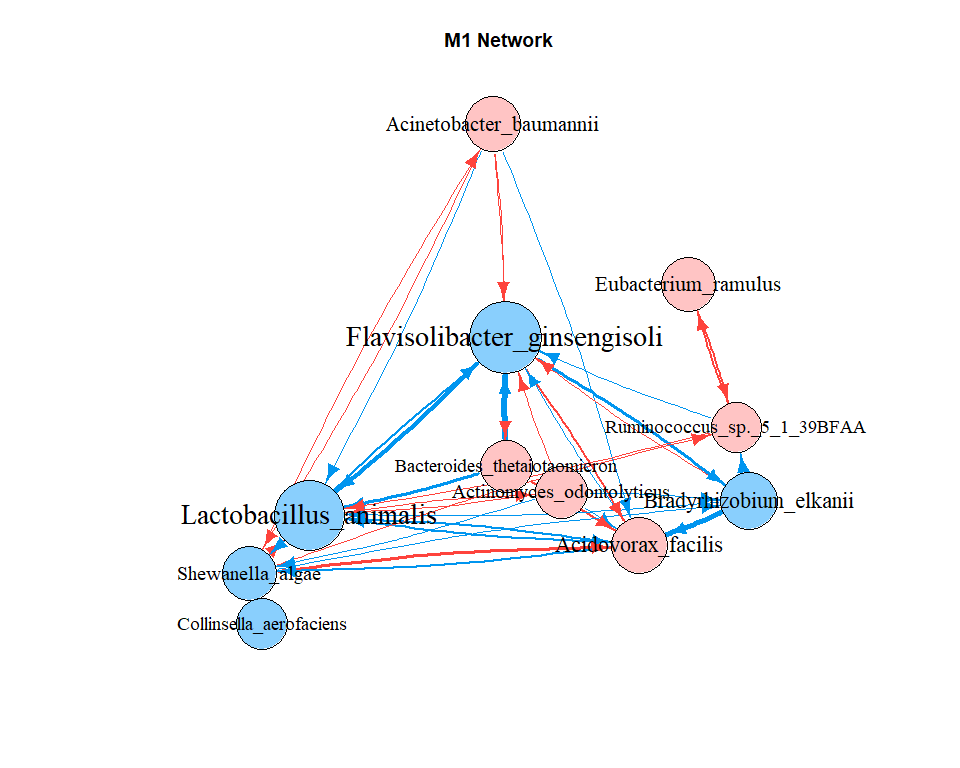## 6.3 network comparison

mustard_module_a = qdODE_parallel(res3$a) mustard_module_b = qdODE_parallel(res3$b)

res_m1a = fun_clu_select(result_fit = res1$dataset1, result_funclu = res3$a, i = 3)
res_m1b = fun_clu_select(result_fit = res1$dataset2, result_funclu = res3$b, i = 3)
mustard_M1a = qdODE_parallel(res_m1a)
mustard_M1b = qdODE_parallel(res_m1b)
mustard_m_a <- lapply(mustard_module_a$ode_result, network_conversion) mustard_m_b <- lapply(mustard_module_b$ode_result, network_conversion)

#set seed to make same random layout
layout(matrix(c(1,2),1,2,byrow=TRUE))
set.seed(1)
network_plot(mustard_m_a, title = "Module Network a")
set.seed(1)
network_plot(mustard_m_b, title = "Module Network b")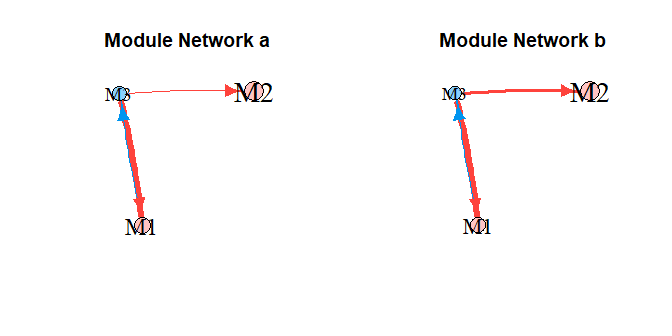result_m1a = fun_clu_select(result_fit = res1$dataset1, result_funclu = res3$a, i = 1)
result_m1b = fun_clu_select(result_fit = res1$dataset2, result_funclu = res3$b, i = 1)
ode.m1a = qdODE_parallel(result_m1a, thread = 16)
ode.m1b = qdODE_parallel(result_m1b, thread = 16)
net_m1a = lapply(ode.m1a$ode_result, network_conversion) net_m1b = lapply(ode.m1b$ode_result, network_conversion)

#set seed to make same random layout
layout(matrix(c(1,2),1,2,byrow=TRUE))
set.seed(1)
network_plot(net_m1a, title = "Module1 a")
set.seed(1)
network_plot(net_m1b, title = "Module1 b")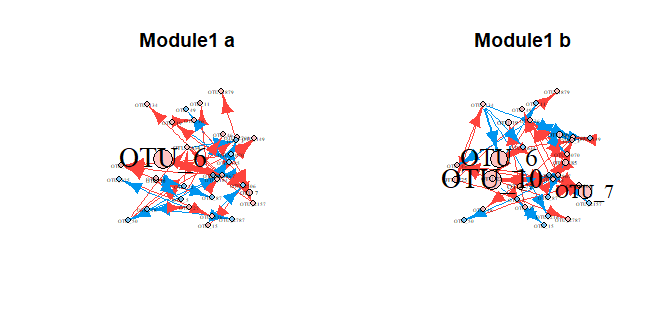# Troubleshooting

This happens when parameters optimization failure, try rerun cluster.

plot failure when using fun_clu_plot() or bifun_clu_plot()
This often happens when bad initial parameters is given and some cluster is lost, try rerun cluster or use a smaller k.

# Session info

sessionInfo()
#> R version 4.2.1 (2022-06-23 ucrt)
#> Platform: x86_64-w64-mingw32/x64 (64-bit)
#> Running under: Windows 10 x64 (build 22000)
#>
#> Matrix products: default
#>
#> locale:
#>  LC_COLLATE=C
#>  LC_CTYPE=English_United States.utf8
#>  LC_MONETARY=English_United States.utf8
#>  LC_NUMERIC=C
#>  LC_TIME=English_United States.utf8
#>
#> attached base packages:
#>  stats     graphics  grDevices utils     datasets  methods   base
#>
#> other attached packages:
#>  idopNetwork_0.1.1
#>
#> loaded via a namespace (and not attached):
#>   deSolve_1.33         shape_1.4.6          tidyselect_1.1.2
#>   xfun_0.33            bslib_0.4.0          reshape2_1.4.4
#>   purrr_0.3.4          splines_4.2.1        lattice_0.20-45
#>  colorspace_2.0-3     vctrs_0.4.2          generics_0.1.3
#>  htmltools_0.5.3      yaml_2.3.5           utf8_1.2.2
#>  survival_3.3-1       rlang_1.0.6          orthopolynom_1.0-6.1
#>  jquerylib_0.1.4      pillar_1.8.1         glue_1.6.2
#>  DBI_1.1.3            plyr_1.8.7           foreach_1.5.2
#>  lifecycle_1.0.3      stringr_1.4.1        munsell_0.5.0
#>  gtable_0.3.1         mvtnorm_1.1-3        codetools_0.2-18
#>  evaluate_0.16        labeling_0.4.2       knitr_1.40
#>  fastmap_1.1.0        parallel_4.2.1       fansi_1.0.3
#>  highr_0.9            Rcpp_1.0.9           polynom_1.4-1
#>  scales_1.2.1         cachem_1.0.6         jsonlite_1.8.2
#>  farver_2.1.1         ggplot2_3.3.6        digest_0.6.29
#>  stringi_1.7.8        dplyr_1.0.10         grid_4.2.1
#>  cli_3.4.1            tools_4.2.1          magrittr_2.0.3
#>  sass_0.4.2           patchwork_1.1.2      glmnet_4.1-4
#>  tibble_3.1.8         pkgconfig_2.0.3      Matrix_1.5-1
#>  assertthat_0.2.1     rmarkdown_2.16       rstudioapi_0.14
#>  iterators_1.0.14     R6_2.5.1             igraph_1.3.5
#>  compiler_4.2.1